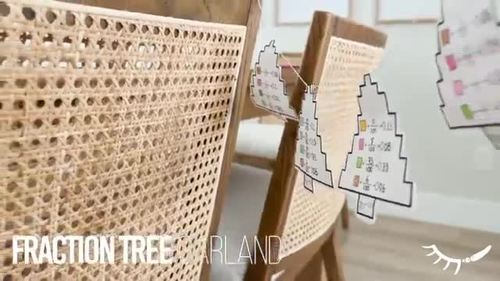# Converting Fractions to Decimals │Paper Garland Math Craft

Rated 4.75 out of 5, based on 4 reviews
4 Ratings;
4th - 6th, Homeschool
Subjects
Resource Type
Standards
Formats Included
• PDF
Pages
9 pages
Report this resource to TPT

### Description

A Winter Math Activity to practice converting fractions to decimals or decimals to fractions. This is a great fractions to decimals worksheet for reviewing decimals in 5th grade, or as a 4th grade Christmas math worksheet to explore as a class.

These paper garlands turn out super cute. Each student can make their own mini tree garland with 3 or more trees, or print out the large blank template and make a tree garland as a class!

How the Christmas Math Activity Works:

Each fractions to decimals worksheet comes with 3 trees per page for students to create their designs using 4 colors. Next students represent the number of squares colored in each color as a fraction and then convert it to a decimal value.

What's Included in this decimal and Fraction Worksheet:

-9 Different templates

-Photo Instructions

(Video Instructions are included on youtube channel)

9 Template Variations:

Templates vary depending on learning objectives.

Pg. 6-8: Blank tree template with either 1, 2, or 3 trees per page.

Pg. 9-10: Tree template comes with predetermined fractions with a denominator of 100 for students to convert to decimals.

Pg. 11-12: Tree template comes with predetermined fractions of varying denominator values for students to convert to equivalent decimal values.

Pg. 13-14: Tree template comes with predetermined decimal values for students to convert to equivalent fraction values.

Connect with Me:

I would also LOVE to see how you use them in your classroom! Feel free to tag me or send me a message if you have any questions.

New products are posted at a discount for the first 48 hours! Follow me to know about sales and new product releases.

instagram: @draw.calm

Total Pages
9 pages
N/A
Teaching Duration
1 hour
Report this resource to TPT
Reported resources will be reviewed by our team. Report this resource to let us know if this resource violates TPT’s content guidelines.

### Standards

to see state-specific standards (only available in the US).
Explain patterns in the number of zeros of the product when multiplying a number by powers of 10, and explain patterns in the placement of the decimal point when a decimal is multiplied or divided by a power of 10. Use whole-number exponents to denote powers of 10.
Add, subtract, multiply, and divide decimals to hundredths, using concrete models or drawings and strategies based on place value, properties of operations, and/or the relationship between addition and subtraction; relate the strategy to a written method and explain the reasoning used.
Explain why a fraction 𝘢/𝘣 is equivalent to a fraction (𝘯 × 𝘢)/(𝘯 × 𝘣) by using visual fraction models, with attention to how the number and size of the parts differ even though the two fractions themselves are the same size. Use this principle to recognize and generate equivalent fractions.
Understand a fraction 𝘢/𝘣 with 𝘢 > 1 as a sum of fractions 1/𝘣.
Understand addition and subtraction of fractions as joining and separating parts referring to the same whole.